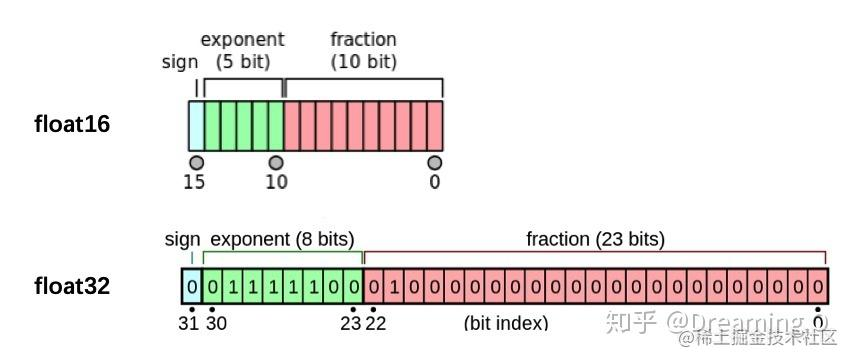# Pytorch學習（六）——

## 6.1 自定義損失函數

PyTorch在torch.nn模塊為我們提供了許多常用的損失函數，比如：MSELoss，L1Loss，BCELoss...... 但是隨着深度學習的發展，出現了越來越多的非官方提供的Loss，比如DiceLoss，HuberLoss，SobolevLoss...... 這些Loss Function專門針對一些非通用的模型，PyTorch不能將他們全部添加到庫中去，因此這些損失函數的實現則需要我們通過自定義損失函數來實現。另外，在科學研究中，我們往往會提出全新的損失函數來提升模型的表現，這時我們既無法使用PyTorch自帶的損失函數，也沒有相關的博客供參考，此時自己實現損失函數就顯得更為重要了。

### 6.1.1 以函數方式定義

python def my_loss(output, target): loss = torch.mean((output - target)**2) return loss

### 6.1.2 以類方式定義

Dice Loss是一種在分割領域常見的損失函數，定義如下：

$$DSC = \frac{2|X∩Y|}{|X|+|Y|}$$ 實現代碼如下：

python class DiceLoss(nn.Module): def init(self,weight=None,size_average=True): super(DiceLoss,self).init()

def forward(self,inputs,targets,smooth=1):
inputs = F.sigmoid(inputs)
inputs = inputs.view(-1)
targets = targets.view(-1)
intersection = (inputs * targets).sum()
dice = (2.*intersection + smooth)/(inputs.sum() + targets.sum() + smooth)
return 1 - dice


# 使用方法

criterion = DiceLoss() loss = criterion(input,targets) 

python class DiceBCELoss(nn.Module): def init(self, weight=None, size_average=True): super(DiceBCELoss, self).init()

def forward(self, inputs, targets, smooth=1):
inputs = F.sigmoid(inputs)
inputs = inputs.view(-1)
targets = targets.view(-1)
intersection = (inputs * targets).sum()
dice_loss = 1 - (2.*intersection + smooth)/(inputs.sum() + targets.sum() + smooth)
BCE = F.binary_cross_entropy(inputs, targets, reduction='mean')
Dice_BCE = BCE + dice_loss

return Dice_BCE


class IoULoss(nn.Module): def init(self, weight=None, size_average=True): super(IoULoss, self).init()

def forward(self, inputs, targets, smooth=1):
inputs = F.sigmoid(inputs)
inputs = inputs.view(-1)
targets = targets.view(-1)
intersection = (inputs * targets).sum()
total = (inputs + targets).sum()
union = total - intersection

IoU = (intersection + smooth)/(union + smooth)

return 1 - IoU


ALPHA = 0.8 GAMMA = 2

class FocalLoss(nn.Module): def init(self, weight=None, size_average=True): super(FocalLoss, self).init()

def forward(self, inputs, targets, alpha=ALPHA, gamma=GAMMA, smooth=1):
inputs = F.sigmoid(inputs)
inputs = inputs.view(-1)
targets = targets.view(-1)
BCE = F.binary_cross_entropy(inputs, targets, reduction='mean')
BCE_EXP = torch.exp(-BCE)
focal_loss = alpha * (1-BCE_EXP)**gamma * BCE

return focal_loss


# 更多的可以參考鏈接1



### 本節參考

【1】https://www.kaggle.com/bigironsphere/loss-function-library-keras-pytorch/notebook
【3】https://blog.csdn.net/dss_dssssd/article/details/84103834
【5】https://blog.csdn.net/qq_27825451/article/details/95165265
【6】https://discuss.pytorch.org/t/should-i-define-my-custom-loss-function-as-a-class/89468

## 6.2 動態調整學習率

### 6.2.1 使用官方scheduler

• 瞭解官方提供的API

python

# 選擇上面提到的一種或多種動態調整學習率的方法

scheduler1 = torch.optim.lr_scheduler.... scheduler2 = torch.optim.lr_scheduler.... ... schedulern = torch.optim.lr_scheduler....

# 進行訓練

for epoch in range(100): train(...) validate(...) optimizer.step() # 需要在優化器參數更新之後再動態調整學習率 scheduler1.step() ... schedulern.step() 

### 6.2.2 自定義scheduler

python def adjust_learning_rate(optimizer, epoch): lr = args.lr * (0.1 ** (epoch // 30)) for param_group in optimizer.param_groups: param_group['lr'] = lr

python def adjust_learning_rate(optimizer,...): ... optimizer = torch.optim.SGD(model.parameters(),lr = args.lr,momentum = 0.9) for epoch in range(10): train(...) validate(...) adjust_learning_rate(optimizer,epoch)

【1】PyTorch官方文檔

## 6.3 模型微調

### 6.3.1 模型微調的流程

1. 在源數據集(如ImageNet數據集)上預訓練一個神經網絡模型，即源模型。
2. 創建一個新的神經網絡模型，即目標模型。它複製了源模型上除了輸出層外的所有模型設計及其參數。我們假設這些模型參數包含了源數據集上學習到的知識，且這些知識同樣適用於目標數據集。我們還假設源模型的輸出層跟源數據集的標籤緊密相關，因此在目標模型中不予採用。
3. 為目標模型添加一個輸出⼤小為⽬標數據集類別個數的輸出層，並隨機初始化該層的模型參數。
4. 在目標數據集上訓練目標模型。我們將從頭訓練輸出層，而其餘層的參數都是基於源模型的參數微調得到的。### 6.3.2 使用已有模型結構

• 實例化網絡

python import torchvision.models as models resnet18 = models.resnet18() # resnet18 = models.resnet18(pretrained=False) 等價於與上面的表達式 alexnet = models.alexnet() vgg16 = models.vgg16() squeezenet = models.squeezenet1_0() densenet = models.densenet161() inception = models.inception_v3() googlenet = models.googlenet() shufflenet = models.shufflenet_v2_x1_0() mobilenet_v2 = models.mobilenet_v2() mobilenet_v3_large = models.mobilenet_v3_large() mobilenet_v3_small = models.mobilenet_v3_small() resnext50_32x4d = models.resnext50_32x4d() wide_resnet50_2 = models.wide_resnet50_2() mnasnet = models.mnasnet1_0() - 傳遞pretrained參數

python import torchvision.models as models resnet18 = models.resnet18(pretrained=True) alexnet = models.alexnet(pretrained=True) squeezenet = models.squeezenet1_0(pretrained=True) vgg16 = models.vgg16(pretrained=True) densenet = models.densenet161(pretrained=True) inception = models.inception_v3(pretrained=True) googlenet = models.googlenet(pretrained=True) shufflenet = models.shufflenet_v2_x1_0(pretrained=True) mobilenet_v2 = models.mobilenet_v2(pretrained=True) mobilenet_v3_large = models.mobilenet_v3_large(pretrained=True) mobilenet_v3_small = models.mobilenet_v3_small(pretrained=True) resnext50_32x4d = models.resnext50_32x4d(pretrained=True) wide_resnet50_2 = models.wide_resnet50_2(pretrained=True) mnasnet = models.mnasnet1_0(pretrained=True)

1. 通常PyTorch模型的擴展為.pt.pth，程序運行時會首先檢查默認路徑中是否有已經下載的模型權重，一旦權重被下載，下次加載就不需要下載了。

2. 一般情況下預訓練模型的下載會比較慢，我們可以直接通過迅雷或者其他方式去 這裏 查看自己的模型裏面model_urls，然後手動下載，預訓練模型的權重在LinuxMac的默認下載路徑是用户根目錄下的.cache文件夾。在Windows下就是C:\Users\<username>\.cache\torch\hub\checkpoint。我們可以通過使用 torch.utils.model_zoo.load_url()設置權重的下載地址。

3. 如果覺得麻煩，還可以將自己的權重下載下來放到同文件夾下，然後再將參數加載網絡。

python self.model = models.resnet50(pretrained=False) self.model.load_state_dict(torch.load('./model/resnet50-19c8e357.pth'))

1. 如果中途強行停止下載的話，一定要去對應路徑下將權重文件刪除乾淨，要不然可能會報錯。

### 6.3.3 訓練特定層

python def set_parameter_requires_grad(model, feature_extracting): if feature_extracting: for param in model.parameters(): param.requires_grad = False

python import torchvision.models as models

# 凍結參數的梯度

feature_extract = True model = models.resnet18(pretrained=True) set_parameter_requires_grad(model, feature_extract)

# 修改模型

num_ftrs = model.fc.in_features model.fc = nn.Linear(in_features=512, out_features=4, bias=True) 

【1】參數更新
【2】給不同層分配不同的學習率

## 6.4 半精度訓練• 如何在PyTorch中設置半精度訓練
• 使用半精度訓練的注意事項

### 6.4.1 半精度訓練的設置

• import autocast

python from torch.cuda.amp import autocast

• 模型設置

python @autocast() def forward(self, x): ... return x

• 訓練過程

python for x in train_loader: x = x.cuda() with autocast(): output = model(x) ...`

「其他文章」